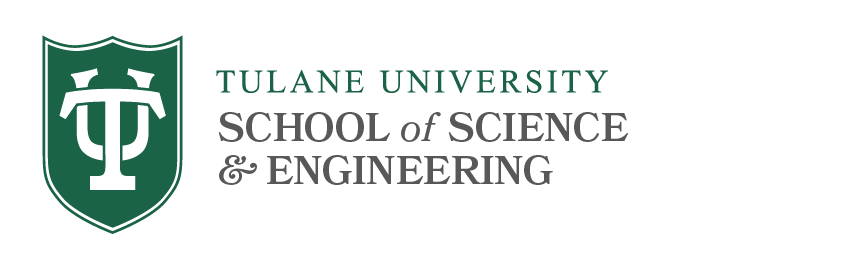# Geometry and Topology 2018 Fall

Fall 2018
Time & Location: All talks are on Thursday in Gibson Hall 400D at 12:30 PM unless otherwise noted.
Organizer: Slawomir Kwasik

October 18
Topic: On the reconstruction problem for geodesic subspaces in the Euclidean Space
Rafał Komendarczyk - Tulane University
Abstract:
We develop a persistence based algorithm for the homology/homotopy groups reconstruction of the unknown underlying geodesic subspace of R^n from a point cloud. In the case of a metric graph, we can output a subspace which is an arbitrarily good approximation of the underlying graph. This is a collaborative work in progress.

October 25
Finite-type knot invariants and a proof of the Goussarov Theorem

Robyn Brooks - Tulane University
Abstract:

Finite-type knot invariants represent an active research area in knot theory.  The Goussarov Theorem shows that all such invariants can be read from the Gauss diagram of a knot.  In this talk, I will give a proof of this theorem, which provides a method by which one can generate a formula to determine the value of an invariant from its Gauss diagram.

November 1
Discrete Morse theory and its applications to metric graph reconstruction

Sushovan Majhi - Tulane University
Abstract:
Discrete Morse theory has recently been used as a powerful tool for topological reconstruction and simplification in the field of Applied and Computational Topology.  We discuss some its very recent and successful applications in data analysis. In particular, we talk about a recent development in threshold-based topological and geometric reconstruction of metric graphs from a density concentrated around it.

November 15
Density bounds on binary packings of disks in the plane

Ali Mohajer - Tulane University
Abstract:

In this talk we develop methods for establishing upper density bounds for saturated two-radius packings of disks in the plane.

Define the homogeneity of a packing of disks in the plane to be the infimum of the ratio of radii of disks in the packing. It has been known since 1953 (L. Fejes-Toth) that if the homogeneity of a packing is close enough to 1, the density of that packing cannot exceed $\frac{$\pi}{\sqrt{12}},\$ the upper bound on the density of a single-radius packing. "Close enough” was refined in 1963 by August Florian to mean a homogeneity in the interval (0.902…, 1], and in 1969, Gerd Blind extended the left bound of this interval to approximately 0.742.

In 2003, sharp upper density bounds were established by Aladar Heppes for a handful of two-radius packings at homogeneities which admit arrangements wherein each disk is tangent to a ring of disks, each of which is tangent to its two cyclic neighbors. We will discuss recent progress in establishing a bound sharper than the best one known for binary packings at a homogeneity that does not admit such regularity.